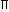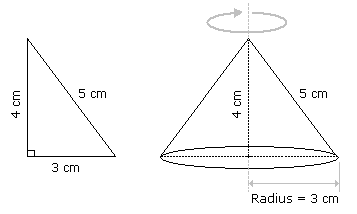# Aptitude - Volume and Surface Area

Exercise : Volume and Surface Area - General Questions
1.
A right triangle with sides 3 cm, 4 cm and 5 cm is rotated the side of 3 cm to form a cone. The volume of the cone so formed is:
12cm3
15cm3
16cm3
20cm3
Explanation:Clearly, we have r = 3 cm and h = 4 cm.Volume = 1r2h =1 xx 32 x 4cm3 = 12cm3. 3 3

Video Explanation: https://youtu.be/Njsid2mVwUY

2.
In a shower, 5 cm of rain falls. The volume of water that falls on 1.5 hectares of ground is:
75 cu. m
750 cu. m
7500 cu. m
75000 cu. m
Explanation:

1 hectare = 10,000 m2

So, Area = (1.5 x 10000) m2 = 15000 m2.

 Depth = 5 m = 1 m. 100 20Volume = (Area x Depth) =15000 x 1m3 = 750 m3. 20

Video Explanation: https://youtu.be/dDbVuBzYop8

3.
A hall is 15 m long and 12 m broad. If the sum of the areas of the floor and the ceiling is equal to the sum of the areas of four walls, the volume of the hall is:
720
900
1200
1800
Explanation:

2(15 + 12) x h = 2(15 x 12)h = 180 m = 20 m. 27 3Volume =15 x 12 x 20m3 = 1200 m3. 3

Video Explanation: https://youtu.be/V8EQ1YIaH74

4.
66 cubic centimetres of silver is drawn into a wire 1 mm in diameter. The length of the wire in metres will be:
84
90
168
336
Explanation:

Let the length of the wire be h.

 Radius = 1 mm = 1 cm. Then, 2 2022 x 1 x 1 x h = 66. 7 20 20h =66 x 20 x 20 x 7= 8400 cm = 84 m. 22

5.
A hollow iron pipe is 21 cm long and its external diameter is 8 cm. If the thickness of the pipe is 1 cm and iron weighs 8 g/cm3, then the weight of the pipe is:
3.6 kg
3.696 kg
36 kg
36.9 kg
 =22 x [(4)2 - (3)2] x 21cm3 7
 =22 x 7 x 1 x 21cm3 7Weight of iron = (462 x 8) gm = 3696 gm = 3.696 kg.